# Amihood Amir Avivit Levy Ely Porat and B

• Slides: 26Amihood Amir, Avivit Levy, Ely Porat and B. Riva Shalom CPM 2014 1 DICTIONARY MATCHING WITH ONE GAPCPM 2014 - MOSCOW CPM 2014 2MIND THE GAP! CPM 2014 3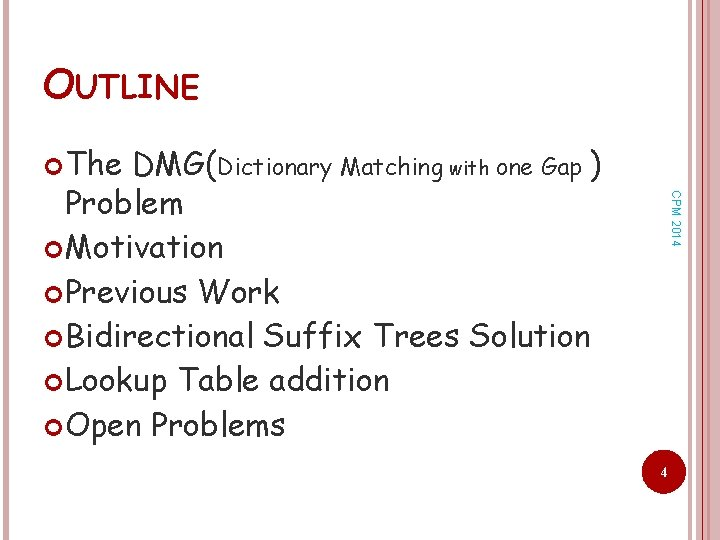OUTLINE The CPM 2014 DMG(Dictionary Matching with one Gap ) Problem Motivation Previous Work Bidirectional Suffix Trees Solution Lookup Table addition Open Problems 4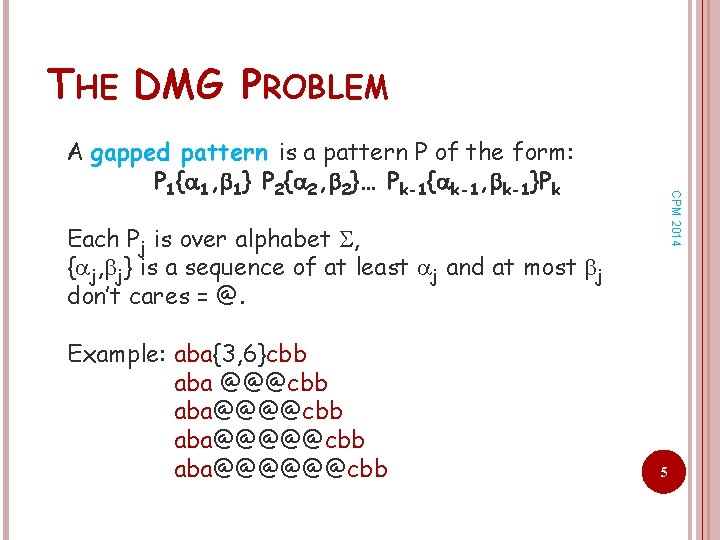THE DMG PROBLEM CPM 2014 A gapped pattern is a pattern P of the form: P 1{ 1, 1} P 2{ 2, 2}… Pk-1{ k-1, k-1}Pk Each Pj is over alphabet , { j, j} is a sequence of at least j and at most j don’t cares = @. Example: aba{3, 6}cbb aba @@@cbb [email protected]@@@@cbb [email protected]@@@@@cbb 5THE DMG PROBLEM The DMG problem is: Preprocess: A dictionary D of d gapped CPM 2014 patterns P 1, …, Pd over alphabet . Query: A text T of length n over alphabet . Output: all locations in T where a dictionary gapped pattern ends. We focus on DMG with a single gap. 6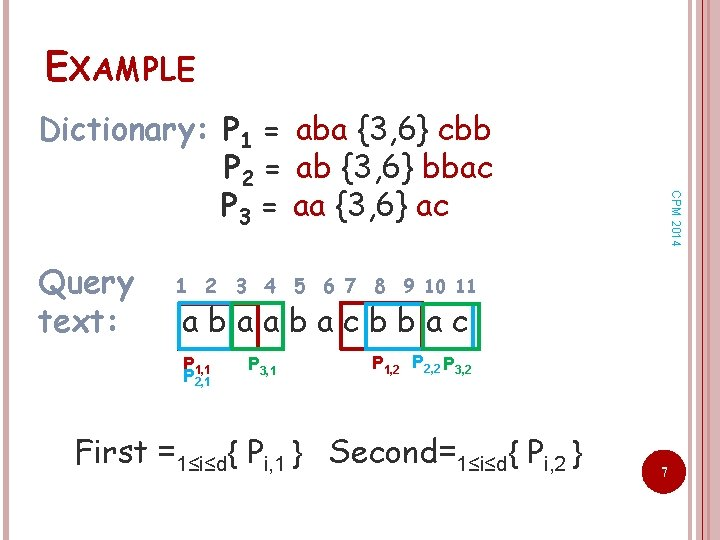EXAMPLE Query text: CPM 2014 Dictionary: P 1 = aba {3, 6} cbb P 2 = ab {3, 6} bbac P 3 = aa {3, 6} ac 1 2 3 4 5 6 7 8 9 10 11 abaabacbbac P 1, 1 P 2, 1 P 3, 1 P 1, 2 P 2, 2 P 3, 2 First =1≤i≤d{ Pi, 1 } Second=1≤i≤d{ Pi, 2 } 7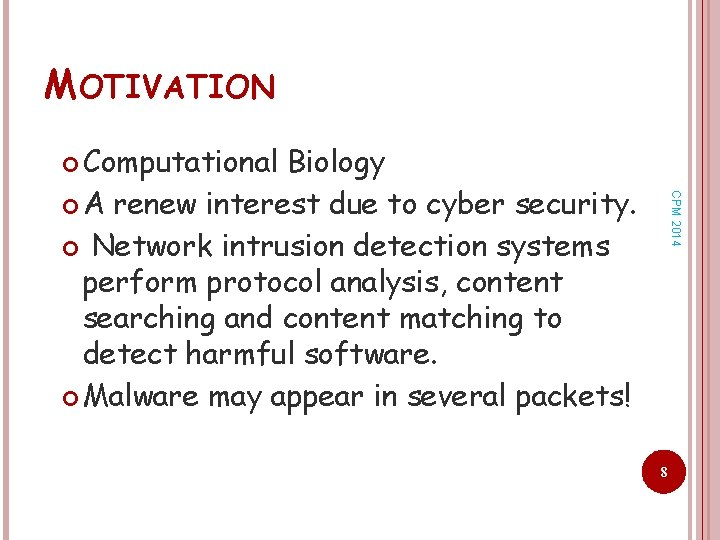MOTIVATION Computational CPM 2014 Biology A renew interest due to cyber security. Network intrusion detection systems perform protocol analysis, content searching and content matching to detect harmful software. Malware may appear in several packets! 8PREVIOUS WORK Gapped CPM 2014 pattern matching problem was studied for a few decades, eg. [Myers, JACM 1992], [Navaro&Raffinot, Algorithmica 2004], [Bille&Thorup, ICALP 2009] , [Bille&Thorup SODA 2010], [Morgante et al. , JCB 2005], [Rahman et al. , COCOON 2006], [Bille et al. , TCS 2012] DMG problem not studied enough ! [Kucherov&Rosinovich, TCS 1997], [Zhang et al. , IPL 2010]-no bounds on the length of the gap. 9BI-DIRECTIONAL SUFFIX TREES ALGORITHM Query: CPM 2014 Gapped pattern: a b{3, 6}b b a c abaabacbbac 10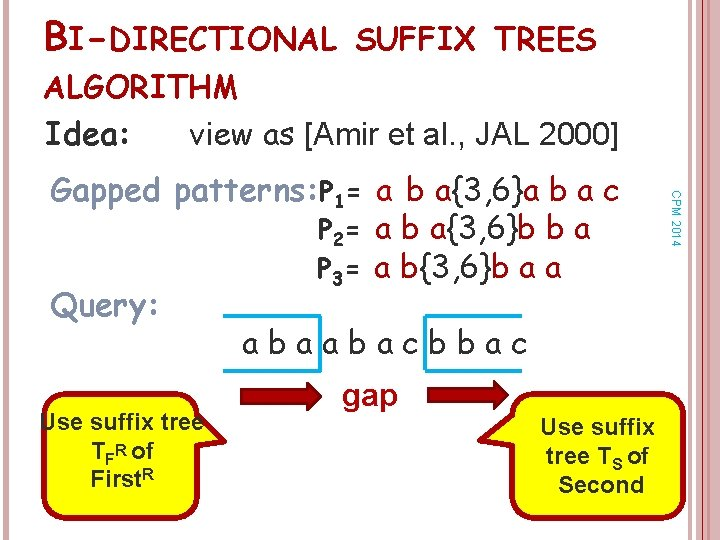BI-DIRECTIONAL SUFFIX TREES ALGORITHM Idea: view as [Amir et al. , JAL 2000] Use suffix tree TFR of First. R CPM 2014 Gapped patterns: P 1= a b a{3, 6}a b a c P 2= a b a{3, 6}b b a P 3= a b{3, 6}b a a Query: abaabacbbac gap Use suffix tree TS of 11 SecondBI-DIRECTIONAL SUFFIX TREES ALGORITHM Finds Pi, 2 starting at location l. CPM 2014 For each text location l Insert tl tl +1…tn to TS (the node h) to find labels on the path to h. For f= l - -1 to l - -1 Insert tftf-1…t 1 to TFR (the node g) to find labels on the path to g. Finds Pi, 1 ending at location f. Output intersection (for end locations). 12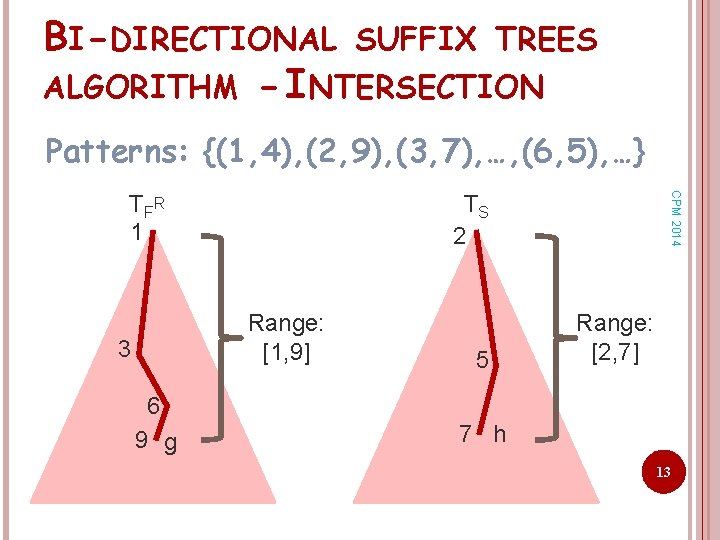BI-DIRECTIONAL SUFFIX TREES ALGORITHM - INTERSECTION Patterns: {(1, 4), (2, 9), (3, 7), …, (6, 5), …} TS 2 Range: [1, 9] 3 6 9 g CPM 2014 T FR 1 5 Range: [2, 7] 7 h 13BI-DIRECTIONAL SUFFIX TREES ALGORITHM (CONTINUED) Intersection via range queries: (2, 9) CPM 2014 (8, 8) (3, 7) (6, 5) Range: [2, 7] (1, 4) 14 Range: [1, 9]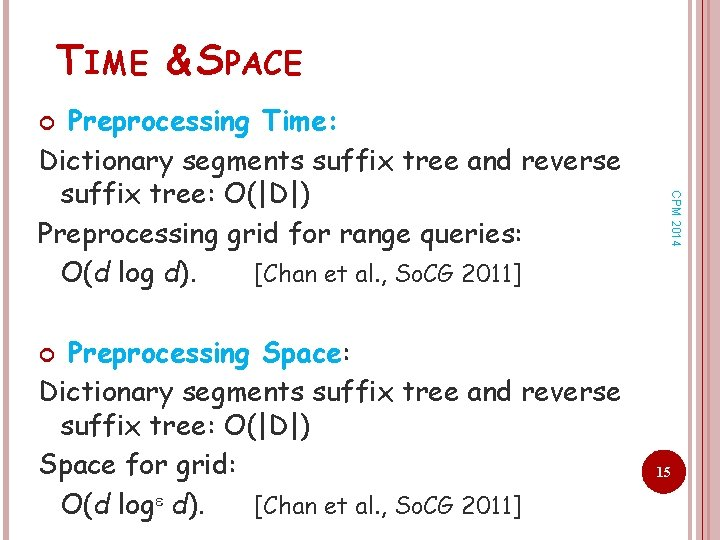TIME & SPACE Preprocessing Time: Dictionary segments suffix tree and reverse suffix tree: O(|D|) Preprocessing grid for range queries: O(d log d). [Chan et al. , So. CG 2011] CPM 2014 Preprocessing Space: Dictionary segments suffix tree and reverse suffix tree: O(|D|) Space for grid: O(d log d). [Chan et al. , So. CG 2011] 15TIME & SPACE Query Time: For each end text location, we try every gap size: a factor of . The number of range queries is the number of vertical paths in a given path: 1 O(log 2 min{d, log |D|}). A range query costs: O(log d+occ). 3 CPM 2014 [Chan et al. , So. CG 2011] Total: O(n( )log d log 2 min{d, log |D|}+occ). 6 9 g 16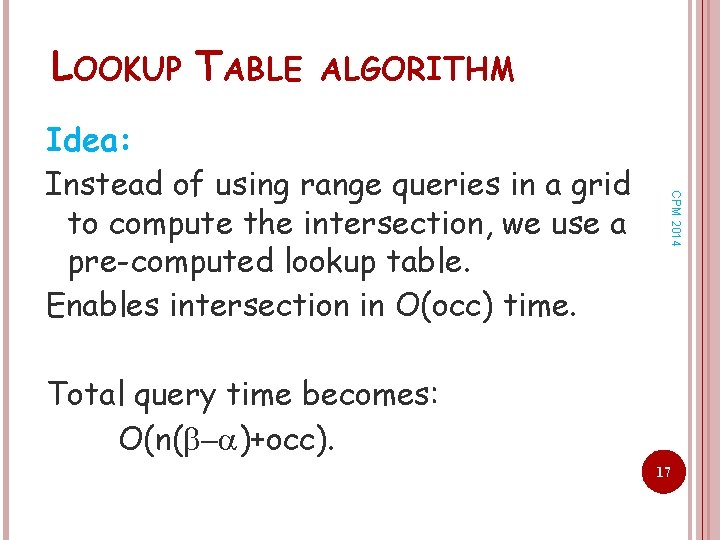LOOKUP TABLE ALGORITHM CPM 2014 Idea: Instead of using range queries in a grid to compute the intersection, we use a pre-computed lookup table. Enables intersection in O(occ) time. Total query time becomes: O(n( )+occ). 17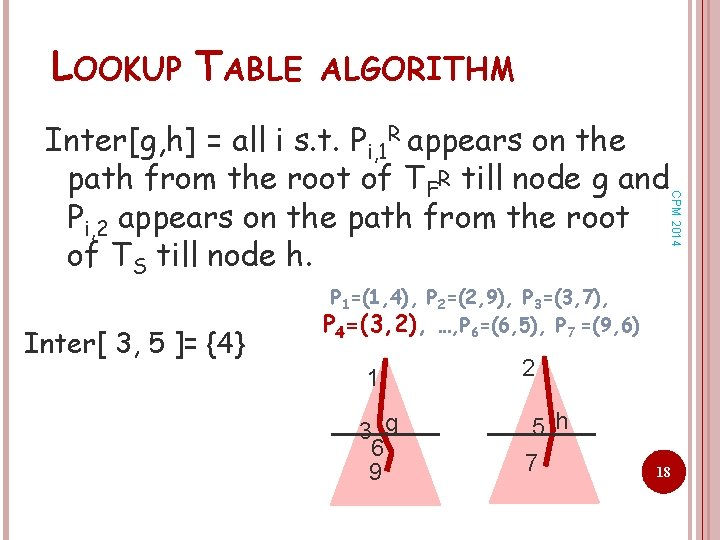LOOKUP TABLE ALGORITHM CPM 2014 Inter[g, h] = all i s. t. Pi, 1 R appears on the path from the root of TFR till node g and Pi, 2 appears on the path from the root of TS till node h. Inter[ 3, 5 ]= {4} P 1=(1, 4), P 2=(2, 9), P 3=(3, 7), P 4=(3, 2), …, P 6=(6, 5), P 7 =(9, 6) 1 3 g 6 9 2 5 h 7 18LOOKUP TABLE ALGORITHM CPM 2014 Inter[g, h] = all i s. t. Pi, 1 R appears on the path from the root of TFR till node g and Pi, 2 appears on the path from the root of TS till node h. Inter[ 3, 5 ]= {4} Inter[ 3, 7 ]= {3, 4} P 1=(1, 4), P 2=(2, 9), P 3=(3, 7), P 4=(3, 2), …, P 6=(6, 5), P 7 =(9, 6) 1 3 g 6 9 2 5 7 h 19LOOKUP TABLE ALGORITHM CPM 2014 Inter[g, h] = all i s. t. Pi, 1 R appears on the path from the root of TFR till node g and Pi, 2 appears on the path from the root of TS till node h. Inter[ 3, 5 ]= {4} Inter[ 3, 7 ]= {3, 4} Inter[ 6, 7 ]= {3, 4, 6} P 1=(1, 4), P 2=(2, 9), P 3=(3, 7), P 4=(3, 2), …, P 6=(6, 5), P 7 =(9, 6) 1 3 g 6 9 2 5 7 h 20LOOKUP TABLE ALGORITHM CPM 2014 Inter[g, h] = all i s. t. Pi, 1 R appears on the path from the root of TFR till node g and Pi, 2 appears on the path from the root of TS till node h. Inter[ 3, 5 ]= {4} Inter[ 3, 7 ]= {3, 4} Inter[ 6, 7 ]= {3, 4, 6} Inter[ 9, 7 ]= {3, 4, 6} P 1=(1, 4), P 2=(2, 9), P 3=(3, 7), P 4=(3, 2), …, P 6=(6, 5), P 7 =(9, 6) 1 3 6 9 g 2 5 7 h 21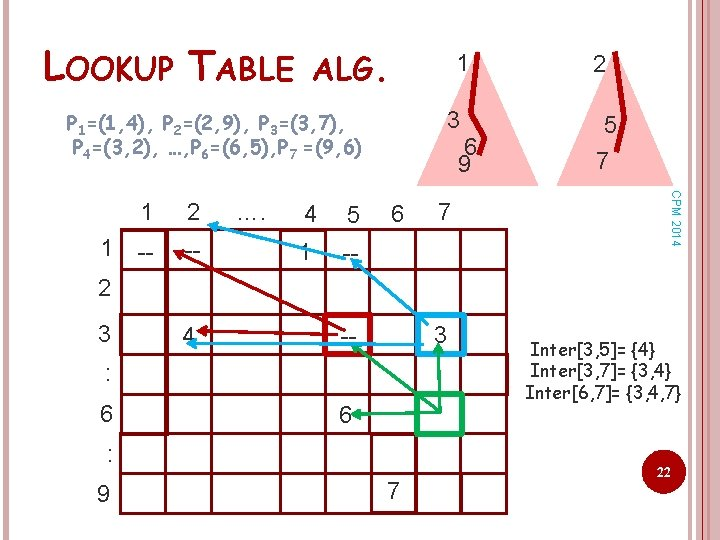LOOKUP TABLE ALG. 1 3 P 1=(1, 4), P 2=(2, 9), P 3=(3, 7), P 4=(3, 2), …, P 6=(6, 5), P 7 =(9, 6) 2 -- -- …. 4 5 1 -- 6 7 5 7 CPM 2014 1 1 6 9 2 2 3 4 3 -- : 6 6 : 9 7 Inter[3, 5]= {4} Inter[3, 7]= {3, 4} Inter[6, 7]= {3, 4, 7} 22LOOKUP TABLE ALGORITHM CPM 2014 Preprocessing: Time: Table can be computed using DP in time O(d 2 ovr + |D|) where ovr is the number of subpatterns including other subpattern as a prefix or suffix. Space: O(d 2 + |D|). Query time: O(n( )+occ). 23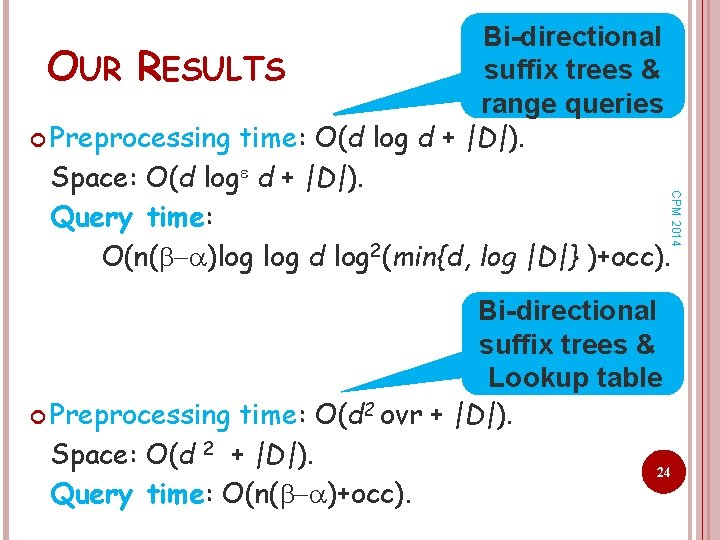CPM 2014 Bi-directional suffix trees & OUR RESULTS range queries Preprocessing time: O(d log d + |D|). Space: O(d log d + |D|). Query time: O(n( )log d log 2(min{d, log |D|} )+occ). Bi-directional suffix trees & Lookup table Preprocessing time: O(d 2 ovr + |D|). Space: O(d 2 + |D|). 24 Query time: O(n( )+occ).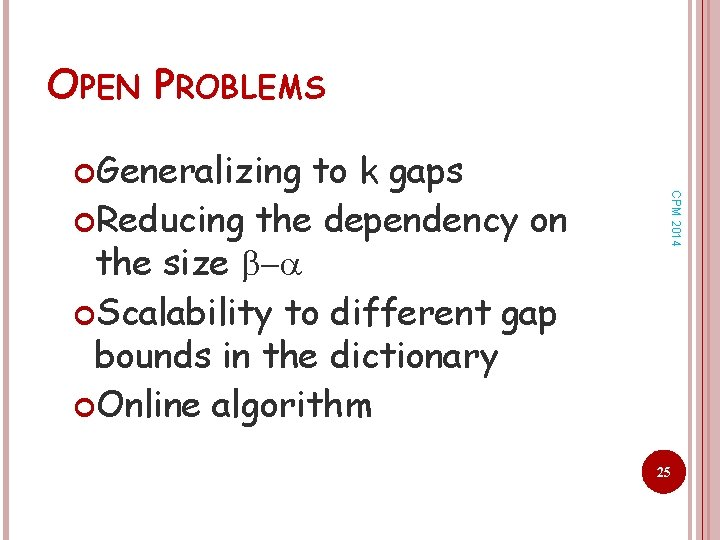OPEN PROBLEMS to k gaps Reducing the dependency on the size Scalability to different gap bounds in the dictionary Online algorithm CPM 2014 Generalizing 25CPM 2014 26